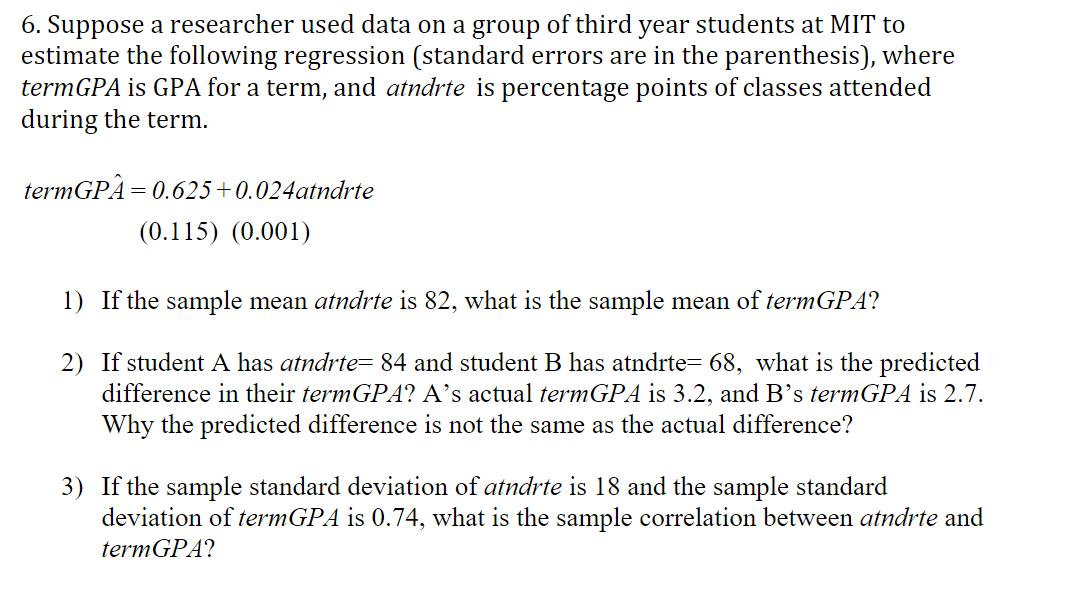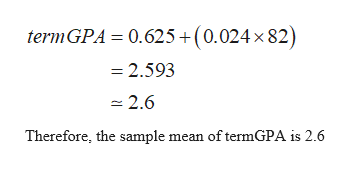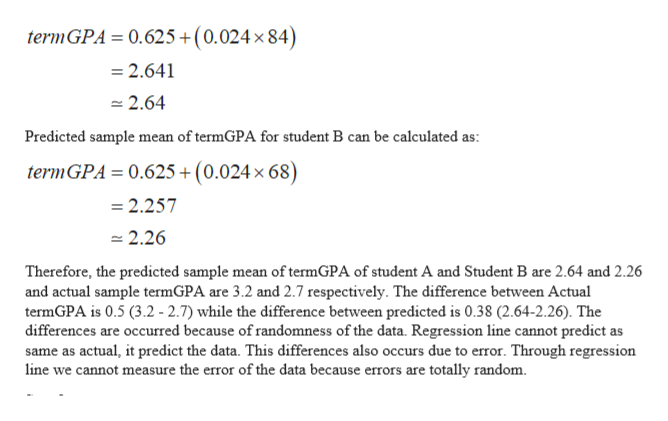# 6. Suppose a researcher used data on a group of third year students at MIT toestimate the following regression (standard errors are in the parenthesis), wheretermGPA is GPA for a term, and atndrte is percentage points of classes attendedduring the termtermGPA 0.625 0.024atndrte(0.115) (0.001)1) If the sample mean atndrte is 82, what is the sample mean of termGPA?2) If student A has atndrte= 84 and student B has atndrte= 68, what is the predicteddifference in their termGPA? A's actual termGPA is 3.2, and B's termGPA is 2.7.Why the predicted difference is not the same as the actual difference?3) If the sample standard deviation of atndrte is 18 and the sample standarddeviation of termGPA is 0.74, what is the sample correlation between atndrte andtermGPA?

Question
18 viewshelp_outlineImage Transcriptionclose6. Suppose a researcher used data on a group of third year students at MIT to estimate the following regression (standard errors are in the parenthesis), where termGPA is GPA for a term, and atndrte is percentage points of classes attended during the term termGPA 0.625 0.024atndrte (0.115) (0.001) 1) If the sample mean atndrte is 82, what is the sample mean of termGPA? 2) If student A has atndrte= 84 and student B has atndrte= 68, what is the predicted difference in their termGPA? A's actual termGPA is 3.2, and B's termGPA is 2.7. Why the predicted difference is not the same as the actual difference? 3) If the sample standard deviation of atndrte is 18 and the sample standard deviation of termGPA is 0.74, what is the sample correlation between atndrte and termGPA? fullscreen
check_circle

Step 1

According to the provided information, the fitted regression line is

Step 2

a)  If the sample mean of atndrte is 82 then the sample mean of termGPA can be calculated as:help_outlineImage Transcriptionclose0.625+(0.024 x 82) termGPA =2.593 2.6 Therefore, the sample mean of termGPA is 2.6 fullscreen
Step 3

b) If student A has 84 atndrte and student B has 68 atndrtet then sample mean of termGPA can be ...help_outlineImage TranscriptionclosetermGPA= 0.625+(0.024 x84) 2.641 2.64 Predicted sample mean of termGPA for student B can be calculated as: term GPA 0.625+(0.024 x 68) =2.257 2.26 Therefore, the predicted sample mean of termGPA of student A and Student B are 2.64 and 2.26 and actual sample termGPA are 3.2 and 2.7 respectively. The difference between Actual termGPA is 0.5 (3.2 - 2.7) while the difference between predicted is 0.38 (2.64-2.26). The differences are occurred because of randomness of the data. Regression line cannot predict as same as actual, it predict the data. This differences also occurs due to error. Through regression line we cannot measure the error of the data because errors are totally random fullscreen

### Want to see the full answer?

See Solution

#### Want to see this answer and more?

Solutions are written by subject experts who are available 24/7. Questions are typically answered within 1 hour.*

See Solution
*Response times may vary by subject and question.
Tagged in

### Statistics